会员中心 | 设为首页 | 加入收藏 | 繁体中文
 网站首页 自考新闻 自考必备 自考风彩 自考课件 资料下载 网上报名 网上答疑 求贤纳士 网校学习首页资源中心机电一体化专业资料1.设随机事件AB互不相容，且P(A)>0P(B)>0，则（D

A.P(A)=1-PB B.P(AB)=P(A)P(B)

C.P(AB)=1 D.P(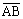)=1

2.AB为随机事件，P(A)>0,PA|B=1，则必有（A

A.P(AB)=P(A) B.A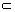B

C.P(A)=P(B) D.P(AB)=P(A)

3.将两封信随机地投入四个邮筒中，则未向前面两个邮筒投信的概率为（A

A.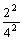B.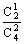C.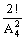D.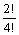4.某人连续向一目标射击，每次命中目标的概率为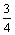，他连续射击直到命中为止，则射击次数为3的概率是（C

A.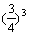B.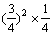C.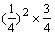D.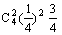5.已知随机变量X的概率密度为fX(x)，令Y=-2X，则Y的概率密度fY(y)为（D

A.2fX(-2y) B.fX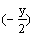C.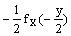D.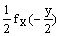6.如果函数

f(x)=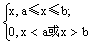A.01 B.02

C.0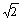D.12

7.下列各函数中是随机变量分布函数的为（B

A.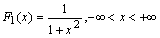B.C.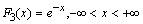D.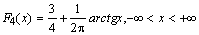8.设二维随机向量（X,Y）的联合分布列为（D

 Y X 0 1 2 0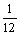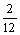10 2P{X=0}=

A.B.C.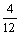D.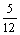9.已知随机变量XY相互独立，且它们分别在区间[-13]上服从均匀分布，则E(XY)=A

A. 3 B. 6

C. 10 D. 12

10.Ф(x)为标准正态分布函数，Xi=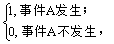i=12…，100，且P(A)=0.8,X1,X2,,X100相互独立。令Y=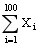，则由中心极限定理知Y的分布函数F(y)近似于（B

A.Ф(y) B.Ф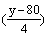C.Ф(16y+80) D.Ф(4y+80)

11.一口袋中装有3只红球，2只黑球，今从中任意取出2只球，则这2只球恰为一红一黑的概率是 0.6   .

12.P(A)=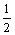P(B|A)=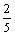，则P(AB)=   0.2    .

13.已知随机变量X的分布列为

 X 1 2 3 4 5 P 2a 0.1 0.3 a 0.3

14.设随机变量XN01），Ф(x)为其分布函数，则Ф(x)+Ф(-x)=   1    .

15.已知连续型随机变量X的分布函数为X的概率密度为f(x)，则当x<0,f(x)=    .

16.设随机变量XY相互独立，且P{X1}=P{Y1}=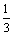，则P{X1Y1}=  1/6    .

17.设随机变量X服从参数为2的泊松分布，则EX2=     6    .

18.设随机变量X的概率密度为f(x)=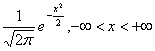，则E(X+1)=    1      .

19.设随机变量XY相互独立，且D(X)=1D(Y)=2，则D(X-Y)=   3     .

20.设随机变量XU[0,1]，由切比雪夫不等式可P{|X-|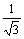}    1/4    .

21.设样本的频数分布为

 X 0 1 2 3 4 频数 1 3 2 1 2

22.设总体XN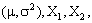,Xn为来自总体X的样本，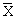为样本均值，则D()=       .

23.设总体X服从正态分布N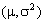，其中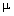未知，X1X2,Xn为其样本。若假设检验问题为H0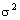=1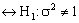，则采用的检验统计量应为       .

24.设某个假设检验问题的拒绝域为W，且当原假设H0成立时，样本值（x1,x2,,xn）落入W的概率为0.15,则犯第一类错误的概率为   0.15

25.设样本X1X2,Xn来自正态总体N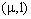，假设检验问题为：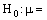0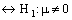，则在H0成立的条件下，对显著水平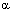，拒绝域W应为       .

26.AB为两个随机事件，0<P(B)<1，且P(A|B)=P(A|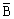)，证明事件AB相互独立。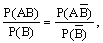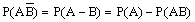AB相互独立。

P(A)=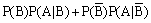=[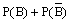]P(A|B)  (由题设)

=P(A|B)

P(AB)=P(B)P(A|B)=P(A)P(B),

AB相互独立。

27.设随机变量X的概率密度为f(x)=E(X)=0.75，求常数c.28.设二维随机向量（XY）的联合概率密度为f(x,y)=1. 求（XY）分别关于XY的边缘概率密度fx(x),fY(y)

2. 判断XY是否相互独立，并说明理由；

3. 计算P{X+Y1}.

解：（1）边缘概率密度为

fx(x)=fx(y)=